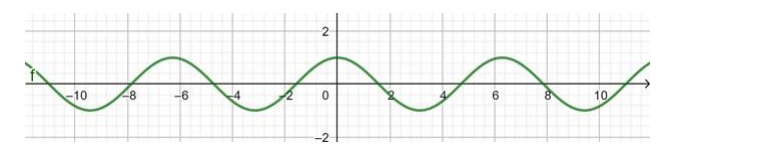# Mark (√) against the correct answer in the following:Question:

Mark (√) against the correct answer in the following:

$f: R \rightarrow R: f(x)=\cos x$ is

A. one - one and into

B. one - one and onto

C. many - one and into

D. many - one and onto

Solution:$f(x)=\cos x$

$y=\cos x$

Here in this range the lines cut the curve in many equal valued points of $y$ therefore the function $f(x)=$ cosx is not one - one.

$\Rightarrow f(x)=$ many one

Range of $f(x)=[-1,1] \neq R$ (codomain)

$\therefore f(x)$ is not onto.

$\Rightarrow f(x)=$ into

Hence, $f(x)=\cos x$ is many one and into

Ans: (c) many - one and into